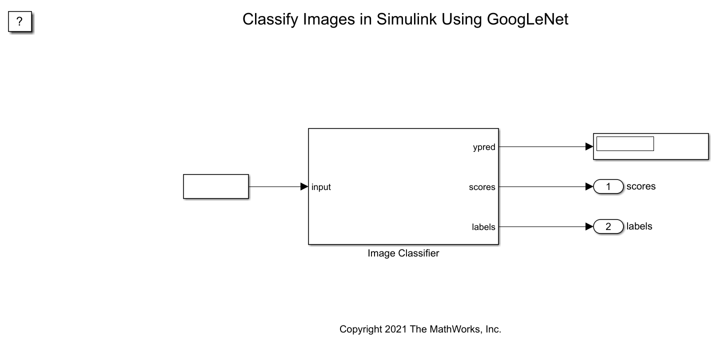This example shows how to classify an image in Simulink® using the Image Classifier block. The example uses the pretrained deep convolutional neural network GoogLeNet to perform the classification.

GoogLeNet has been trained on over a million images and can classify images into 1000 object categories (such as keyboard, coffee mug, pencil, and many animals). The network has learned rich feature representations for a wide range of images. The network takes an image as input, and then outputs a label for the object in the image together with the probabilities for each of the object categories.

inputSize = net.Layers(1).InputSize;
classNames = net.Layers(end).ClassNames;
numClasses = numel(classNames);
disp(classNames(randperm(numClasses,10)))
{'speedboat'    }
{'window screen'}
{'isopod'       }
{'wooden spoon' }
{'lipstick'     }
{'drake'        }
{'hyena'        }
{'dumbbell'     }
{'strawberry'   }
{'custard apple'}

Read and show the image that you want to classify.

figure
imshow(I)To import this data into the Simulink model, specify a structure variable containing the input image data and an empty time vector.

simin.time = [];
simin.signals.values = I;
simin.signals.dimensions = size(I);

The Simulink model for classifying images is shown. The model uses a From Workspace block to load the input image, an Image Classifier block from the Deep Neural Networks library that classifies the input, and Display block to show the predicted output.

open_system(model);Run the Simulation

To validate the Simulink model, run the simulation.

set_param(model,'SimulationMode','Normal');
sim(model);

The network classifies the image as a bell pepper.Display Top Predictions

Display the top five predicted labels and their associated probabilities as a histogram. Because the network classifies images into so many object categories, and many categories are similar, it is common to consider the top-five accuracy when evaluating networks. The network classifies the image as a bell pepper with a high probability.

scores = yout.signals(1).values(:,:,1);
labels = yout.signals(2).values(:,:,1);
[~,idx] = sort(scores,'descend');
idx = idx(5:-1:1);
scoresTop = scores(idx);
labelsTop = split(string(labels(idx)),'_');
labelsTop = labelsTop(:,:,1);

figure
imshow(I)
title(labelsTop(5) + ", " + num2str(100*scoresTop(5) + "%"));

figure
barh(scoresTop)
xlim([0 1])
title('Top 5 Predictions')
xlabel('Probability')
yticklabels(labelsTop)